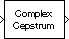# Complex Cepstrum

Compute complex cepstrum of input

•Libraries:
DSP System Toolbox / Transforms

## Description

The Complex Cepstrum block computes the complex cepstrum of the input.

## Ports

### Input

expand all

Specify the input u as a vector or a matrix of size M-by-N. The block treats each column of the input as an independent channel containing M consecutive samples. The block always processes unoriented vector inputs as a single channel, and returns the result as a length-M column vector. The block does not accept complex-valued inputs.

The input is altered by the application of a linear phase term so that there is no phase discontinuity at ±π radians. That is, each input channel is independently zero padded and circularly shifted to have zero phase at π radians.

Data Types: `single` | `double`

### Output

expand all

Complex cepstrum output, returned as an Mo-by-N matrix.

When you clear the Inherit FFT length from input port dimensions parameter, Mo is the value you specify in the FFT length parameter. When you select the Inherit FFT length from input port dimensions parameter, Mo = M, where M is the frame size (number of rows) of the input signal.

Each output column contains the length-Mo complex cepstrum of the corresponding input column.

Here is the equivalent MATLAB® code for computing the complex cepstrum.

`y = cceps(u,Mo)`

where,

• u –– Input

• y –– Complex cepstrum output

• Mo –– Output frame size

The output port rate is the same as the input port rate.

Data Types: `single` | `double`

## Parameters

expand all

When you select this check box, the output frame size Mo matches the input frame size M.

The number of frequency points at which to compute the FFT, which is also the output frame size Mo.

#### Dependency

To enable this parameter, clear the Inherit FFT length from input port dimensions parameter.

## Block Characteristics

 Data Types `double` | `single` Multidimensional Signals `No` Variable-Size Signals `No`

## Version History

Introduced before R2006a